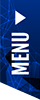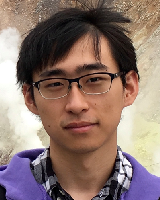# Understanding Modern Machine Learning Models Through the Lens of High-Dimensional Statistics

[CFAR Outstanding PhD Student Seminar Series]
Understanding Modern Machine Learning Models Through the Lens of High-Dimensional Statistics (Hybrid event) by Denny Wu
12 May 2023 | 2.00pm (Singapore Time)

Modern machine learning tasks are often high-dimensional, due to the large amount of data, features, and trainable parameters. Mathematical tools such as random matrix theory have been developed to precisely study simple learning models in the high-dimensional regime, and such precise analysis can reveal interesting phenomena that are also empirically observed in deep learning.

In this talk, Denny Wu from the University of Toronto will introduce two examples concluded by him and his research team. He will first talk about the selection of regularisation hyperparameters in the over-parameterised regime and how the team established a set of equations that rigorously describes the asymptotic generalisation error of the ridge regression estimator. These equations led to some surprising findings: (i) the optimal ridge penalty can be negative and (ii) regularisation can suppress “multiple descents” in the risk curve. He will then discuss practical implications such as the implicit bias of first- and second-order optimisers in neural network training.

Next, Denny will go beyond linear models and characterise the benefit of gradient-based representation (feature) learning in neural networks. By studying the precise performance of kernel ridge regression on the trained features in a two-layer neural network, his team has proven that feature learning could result in a considerable advantage over the initial random features model, highlighting the role of learning rate scaling in the initial phase of gradient descent.

SPEAKERDenny Wu
University of Toronto and the Vector Institute
Denny Wu a Ph.D. student in computer science at the University of Toronto and the Vector Institute, under the supervision of Jimmy Ba and Murat A. Erdogdu. He was previously an undergraduate student at Carnegie Mellon University supervised by Ruslan Salakhutdinov.